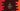# C program find the sum of the harmonic progression## C program find the sum of the harmonic progression or 1 + 1/2 + 1/3 +…n:

In this post, we will learn how to find the sum of the series 1 + 1/2 + 1/3+…n. The base items of this series follows arithmatic progression: 1, 2, 3….

In Arithmatic progression, if the first number is a and common difference is d, the series will be:

``a, a + d, a + 2d, a + 3d....``

It makes easier to find the nth term in an arithmatic progression, which is:

``a + (n - 1)*d``

Similarly, we can find the nth term in a harmonic progression, which is:

``1/(a + (n - 1)*d)``

### C program to find the sum by using a loop:

Let’s try to find the sum of first n numbers in a harmonic progression HP by using a loop. We will write one for loop. This program will read the value of n as input from the user and calculate the harmonic progression value.

Below is the complete program:

``````#include<stdio.h>

double findSum(int n)
{
double sum = 0;

for (double i = 1; i <= n; i++)
{
sum = sum + 1 / i;
}

return sum;
}

int main()
{
int n;
printf("Enter the value of n: ");
scanf("%d", &n);

printf("Sum upto %dth value in HP is: %.2f\n", n, findSum(n));
}``````

Here,

• we are using findSum method to find the sum of first n numbers of the HP.
• This method takes the value of n and returns the value of sum.
• It uses one for loop and cchecks for all values from i = 1 to i = n and finds the sum. This value is returned.

If you run the above program, it will priint output as like below:

``````Enter the value of n: 5
Sum upto 5th value in HP is: 2.28``````

### C program to find harmonic progression recursively:

We can also solve this recursively. A recursive function calls itself with a different argument and calculates the final result.

If we write the above program in recursive, it will look as like below:

``````#include<stdio.h>

double findSum(int n)
{
return n == 1 ? 1 : 1/(double)n + findSum(n - 1);
}

int main()
{
int n;
printf("Enter the value of n: ");
scanf("%d", &n);

printf("Sum upto %dth value in HP is: %.2f\n", n, findSum(n));
}``````

Here,

• findSum is calling itself again and again to find the final sum.
• It will return the same result as the above program.
``````Enter the value of n: 5
Sum upto 5th value in HP is: 2.28``````